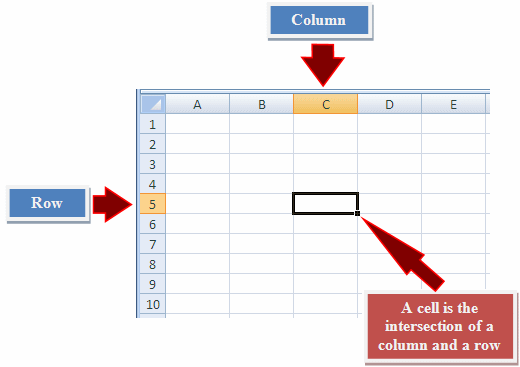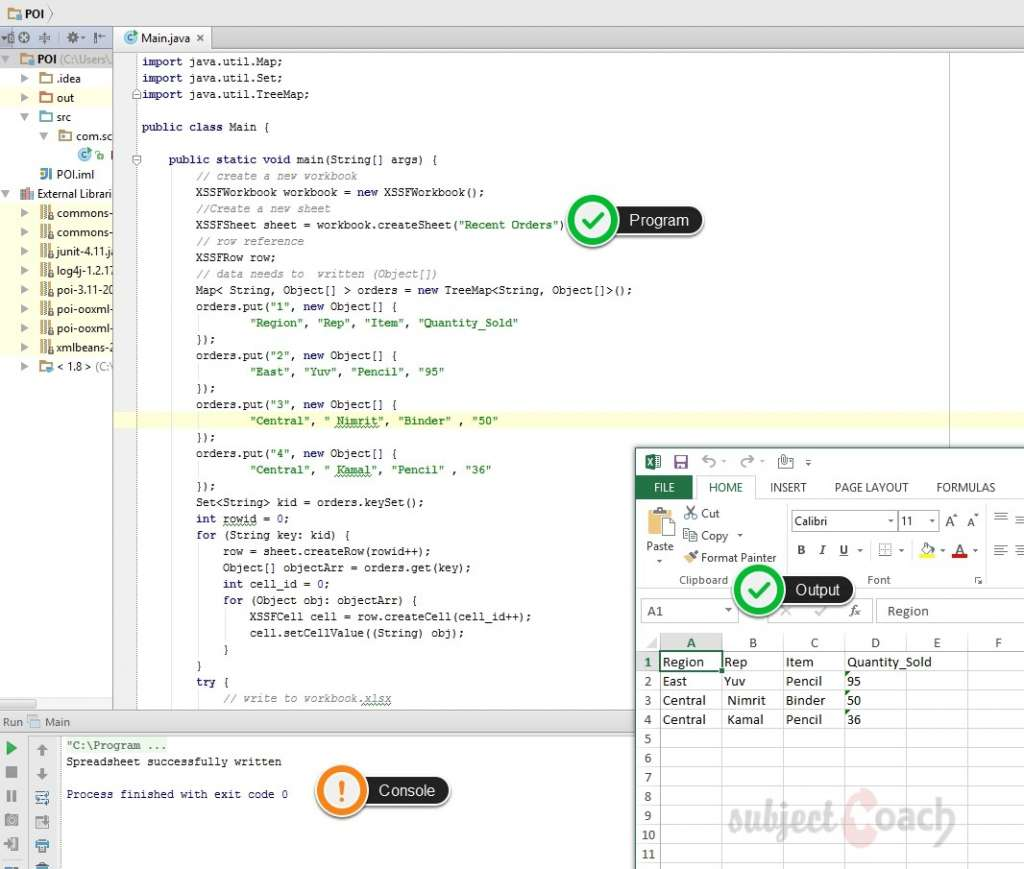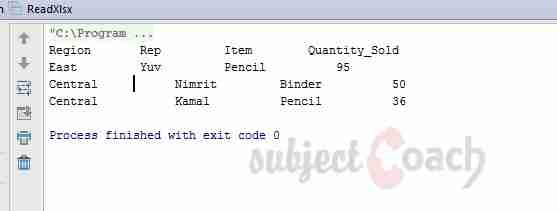# Quick introduction to Apache POI

In this part of the tutorial we will dive into getting to know how to work with spreadsheets, In an excel file, a page is called a Spreadsheet, A spreadsheet always has rows and columns and a referenced by their specific names.

Let's check how to create one.

After creating workbook first, then next step is to create a sheet. Below code can be used to create spreadsheet

Workbook wb = new XSSFWorkbook();
Sheet sheet1 = wb.createSheet("Sheet1");
Sheet sheet2 = wb.createSheet("Sheet2");


There are a few things to note

1. A sheet name in Excel must not exceed 31 characters
2. A sheet name also must not contain any of the any of the these characters:
0x0000, 0x0003, :, \,  *, ?, /, [, ]

Spreadsheets have a grid layout. The columns and rows identified with specific names. The columns identified with alphabets and rows with numbers. An example is shown belowThe following code snippet is used to create a row.

// Example 1 - Create a row in sheet 1. Rows are 0 based.
    Row row = sheet.createRow((short)0);
XSSFRow row = sheet1.createRow((short)1);// Example 2 - Create a row in sheet 1. Rows are 0 based.
XSSFRow row = sheet2.createRow((short)1);

Ok! so we know now how to create a Workbook, a spreadsheet and a row. Let's put this all together and enter some data to our spreadsheet.

Here is some recent order demo data below

 Region Rep Item Quantity_Sold East Yuv Pencil 95 Central Nimrit Binder 50 Central Kamal Pencil 36

Let's get it going with our program below

package com.sc;

import org.apache.poi.xssf.usermodel.XSSFCell;
import org.apache.poi.xssf.usermodel.XSSFRow;
import org.apache.poi.xssf.usermodel.XSSFSheet;
import org.apache.poi.xssf.usermodel.XSSFWorkbook;

import java.io.File;
import java.io.FileOutputStream;
import java.util.Map;
import java.util.Set;
import java.util.TreeMap;

public class Main {

public static void main(String[] args) {
// create a new workbook
XSSFWorkbook workbook = new XSSFWorkbook();
//Create a new sheet
XSSFSheet sheet = workbook.createSheet("Recent Orders");
// row reference
XSSFRow row;
// data needs to  written (Object[])
Map< String, Object[] > orders = new TreeMap<String, Object[]>();
orders.put("1", new Object[] {
"Region", "Rep", "Item", "Quantity_Sold"
});
orders.put("2", new Object[] {
"East", "Yuv", "Pencil", "95"
});
orders.put("3", new Object[] {
"Central", " Nimrit", "Binder" , "50"
});
orders.put("4", new Object[] {
"Central", " Kamal", "Pencil" , "36"
});
Set kid = orders.keySet();
int rowid = 0;
for (String key: kid) {
row = sheet.createRow(rowid++);
Object[] objectArr = orders.get(key);
int cell_id = 0;
for (Object obj: objectArr) {
XSSFCell cell = row.createCell(cell_id++);
cell.setCellValue((String) obj);
}
}
try {
// write to workbook.xlsx
FileOutputStream out = new FileOutputStream(new File("c:/poi/workbook.xlsx"));
workbook.write(out);
out.close();
}catch (Exception e) {
System.out.println(e.getMessage());
}
}
}


Save the above code into Main.java and then compile and run using command prompt as follow:

$javac Main.java$java Main

After compiling the file there will be Excel file in C:\poi directory by file workbook.xlsx.

The Output:Let us consider the above excel file named workbook.xslx as input, following code can be used to read data from the file.

package com.sc;

import org.apache.poi.ss.usermodel.Cell;
import org.apache.poi.ss.usermodel.Row;
import org.apache.poi.xssf.usermodel.XSSFRow;
import org.apache.poi.xssf.usermodel.XSSFSheet;
import org.apache.poi.xssf.usermodel.XSSFWorkbook;

import java.io.File;
import java.io.FileInputStream;
import java.util.Iterator;

static XSSFRow row;
public static void main(String[] args) throws Exception    {
FileInputStream inputStream = new FileInputStream(new File("c:/poi/workbook.xlsx"));
XSSFWorkbook workbook = new XSSFWorkbook(inputStream);
while (rowIterator.hasNext())
{
row = (XSSFRow) rowIterator.next();
Iterator cellIterator = row.cellIterator();
while ( cellIterator.hasNext())
{
Cell cell = cellIterator.next();
switch (cell.getCellType())
{
case Cell.CELL_TYPE_STRING:
System.out.print(
cell.getStringCellValue() + " \t\t " );
break;
case Cell.CELL_TYPE_NUMERIC:
System.out.print(cell.getNumericCellValue() + " \t\t " );
break;
}
}
System.out.println();
}
inputStream.close();

}
}


Save the above code into ReadXlsx.java and then compile and run using command prompt as follow:

$javac ReadXlsx.java$java ReadXlsx

After compiling the file and executing we get the following output. We are using IntelliJ IDE but you get the idea

The output:### Description

This tutorial covers Apache POI, This tutorial is divided into 12 parts as listed below

• What is Apache POI
• Environment
• Core Classes
• Workbooks
• Cells
• Fonts
• Formula
• Hyperlink and defiining Print Area
• Database

Let us know if we made any error, your feedback is important.

### Prerequisites

Its is important that you have working knowledge of Java Programming language

### Audience

Beginners seeking a quick introduction to Apache POI

### Learning Objectives

To get you started with Apache POI

Author: Subject Coach
You must be logged in as Student to ask a Question.